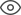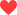Thank you very much for your valuable suggestion! We will solve it as soon as possible!
Community > Groups > Electronics > I-V curves for passive components, voltage sources, and current sources
I-V curves for passive components, voltage sources, and current sources9320158Cash

The current (I) - voltage (V) relationship of electrical components can often provide insight into how electronic devices are used. More specifically, many non-linear devices such as diodes and transistors are used inoperating regionsin which they behave like ideal components—such as current sources, voltage regulators, and resistors.
An understanding of I-V curves often provides insight into knowing how the device operates and helps us know how to operate a device in a way that enables the required functionality.
We'll begin by looking at how to obtain an I-V curve for any component.
Obtaining I-V Curves
Method 1: Voltage Sweeps
The current-voltage (I-V) relationship for a device is a current measured for a given voltage. For devices that do not supply power, I-V curves are obtained by using linear voltage sweeps. Voltage sweeps involve the linear variation of the voltage, to obtain the corresponding measured output current. Because it is impossible to physically sweep through all the voltages in an instant, it is important to understand that these measurements are made with respect to time as well.
It is important to understand that the V vs t information is implicitly present in the I vs V curve. The notion of time is relevant for components that respond to a change in voltage (such as a capacitor) rather than the instantaneous voltage (as with a resistor).
If you have a device that supplies voltage or current, such as a battery or a solar panel or a regular power supply, you cannot change the voltage across the device, because there is a specific voltage or current being generated by the device. For these devices, I-V curves are obtained by load switching.
Load switchingisa method that involves measuring the current supplied by the power source for varying load resistance. The load is the device that electrical power is being delivered to, where power is defined as $$P = V \times I$$.
Typically, a resistor is used as the load to measure the power delivered by a current or a voltage source because they are linear devices that do not exhibit properties of hysteresis, i.e., the operation of the resistor does not depend on its previous state. Because devices can operate with small values of resistance (1-10Ω) as well as large values of resistance (10-1000kΩ), the resistors are varied logarithmically, i.e., from 10 to 100 to 1000 and so on.
This is because a resistor is governed by Ohm's law, and this is an ideal voltage source at a fixed voltage VS; as the resistance value increases logarithmically, the current value decreases logarithmically. Load switching techniques are used to measure the I-V characteristics of devices and circuits that supply power, such as voltage regulator circuits, solar cells, and batteries.
I-V Curves of Ideal Components
Using linear voltage sweeps and load switching, we will now look at the I-V curves of ideal components. In general, if the device requires power to operate, the voltage sweep method is used.
On the other hand, if the device acts as a source of power, the load switching method is used. Based on their basic definitions, we can derive the I-V curves of ideal passive components (resistors, capacitors, and inductors) using the concept of linear voltage sweeps. We will use the concept of load switching for the I-V curves of an ideal voltage source and an ideal current source.
Ideal Resistor
Let's start with one of the more familiar ideal components: the resistor.
The resistor is a component that represents a linear relationship between voltage and current as dictated by Ohm's law, i.e., $$V=I \times R$$.
Ideal Voltage Source
An ideal voltage source is a component that can provide a fixed voltage regardless of the current delivered to the load.
For example, let's say a voltage source supplying 10V is connected across a resistor. If the value of the resistor is $$10k\Omega$$, then the current drawn by the resistor from the voltage supply will be dictated by Ohm's law, which is $$I = \frac{V}{R} = \frac{10V}{10k\Omega} = 1mA$$. If the value of the resistor is 1Ω, then the current drawn will be 10A!
A real voltage supply is limited with respect to the amount of current it can supply for a given voltage, but an ideal voltage source is not. An empirical I-V curve for a real voltage source would be obtained using the load-switching method.
The Zener diode is a non-linear, passive device that is used as a voltage regulator when it is operated in reverse bias. The (idealized) I-V curve of a reverse-biased Zener diode shows that it stays at a particular voltage (determined by the manufacturing process) regardless of the current passing through it. We will be looking at the I-V curve of a Zener diode in a future article.
Ideal Current Source
An ideal current source is a component that can provide a fixed current regardless of the voltage across the component, itself. In other words, a 5A ideal current source would deliver exactly 5A to a 1Ω load resistor or to a 1 kΩ resistor, even though the second resistor would generate a voltage drop of 5000V! This is highly impractical but, nonetheless, ideal current sources are useful tools in circuit analysis.
The I-V curve for an ideal current source is a straight line parallel to the X-axis. An empirical I-V curve for a real current source would be obtained using the load-switching method.
Though "current supplies" are not nearly as common as voltage supplies, many analog transistor circuits are biased using a constant-current source. Also, a MOSFET operating in the saturation region exhibits behavior similar to that of a (voltage-controlled) current source.
Ideal Capacitor
In a resistor, the voltage is determined by the resistance and the current flowing through the resistor. Capacitors and inductors are fundamentally different in that their current-voltage relationships involve the rate of change.
Because we are using a linear voltage sweep, the current through the capacitor is constant when the voltage is increasing or decreasing. When the voltage changes from a positive slope to a negative slope (orange), the direction of the current reverses; this is represented in the current vs. time plot as a change from the positive-current section of the graph to the negative-current section of the graph.
The magnitude of the current is constant, but two horizontal lines are needed because the direction of the current changes depending on whether the voltage is moving from V1 to V2 or V2 to V1. When the voltage has a positive rate of change, the current is positive (indicated by the blue arrowhead); when the voltage has a negative rate of change, the current is negative (indicated by the orange arrowhead).
Ideal Inductor
This means that the current is proportional to the integral of voltage, and this is what we see in the following plots. The current increases in magnitude as the (negative) area under the voltage curve increases. But when the voltage crosses the time axis, the positive area under the curve starts to balance out the negative area under the curve, and this causes the current magnitude to decrease toward zero.
Note the difference between the capacitor and the inductor: With a capacitor, current is proportional to the derivative of voltage, and thus a linear voltage sweep translates to constant current. With an inductor, current is proportional to the integral of voltage, and thus a linear voltage sweep translates to a quadratic shape in the current vs. time plot.
The magnitude of the current gradually increases and then decreases as the voltage moves from V2 to V1 or from V1 to V2. The direction of the current is negative as the voltage moves from V1 to V2 and positive as the voltage moves from V2 to V1.
Summary
The table below summarizes some of the insights we have obtained by looking at the I-V curves of several ideal devices. In a future article, we will look at I-V curves of non-linear devices.
 Device Requires Power? I-V Method Description of the Graph Passes Through Origin? IdealResistor Yes Voltage Sweep Straight line passing through origin Yes IdealVoltage Source No Load Switching Straight line parallel to current axis No IdealCurrent Source No Load Switching Straight line parallel to voltage axis No Ideal Capacitor Yes Voltage Sweep Closed rectangle around origin No Ideal Inductor Yes Voltage Sweep Closed parabolic loop around origin No
158 likes
Statement: This post is only the personal view of the author and does not represent the opinions of ALLPCB.com.Vitor

Marvelous post. Appreciate it very much.